Anzeige

# Chapter5

7. Dec 2017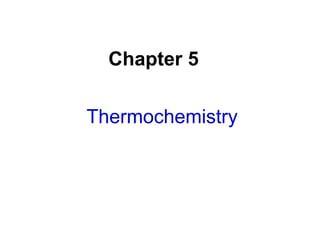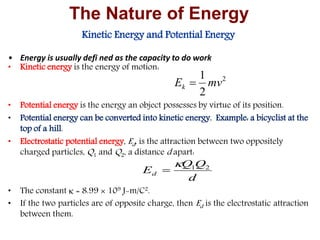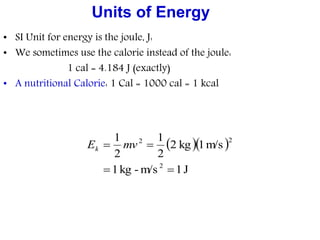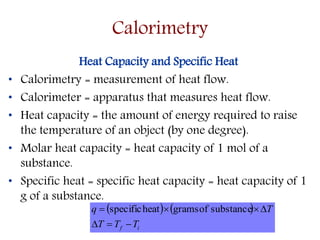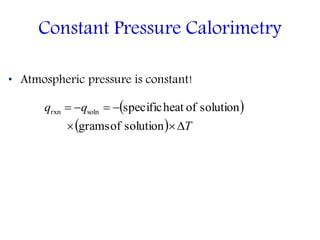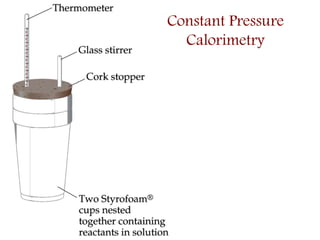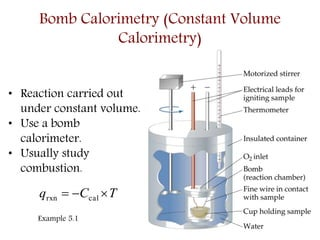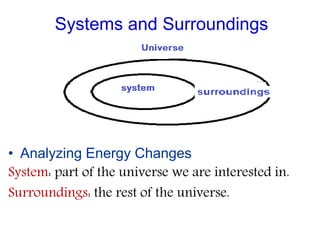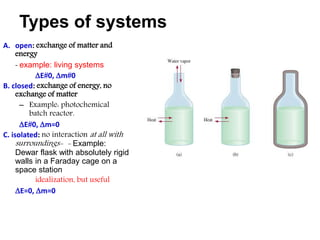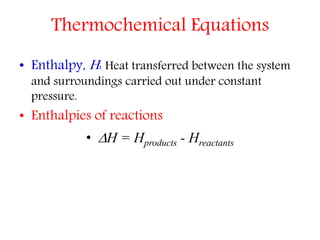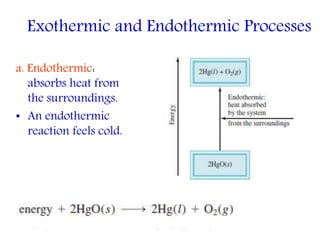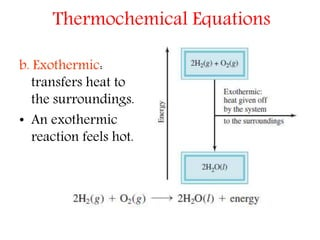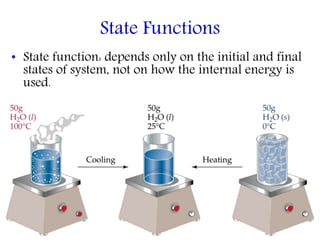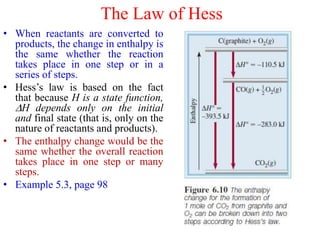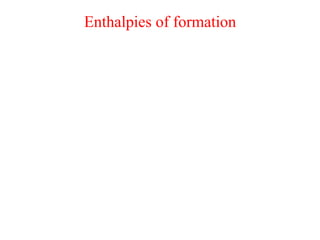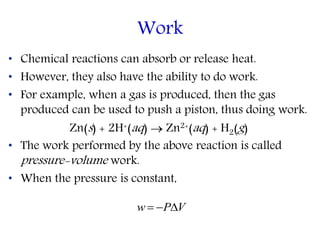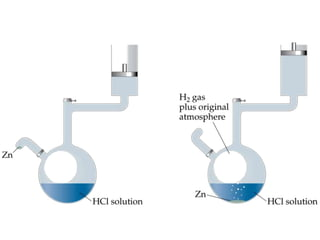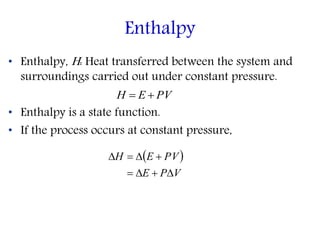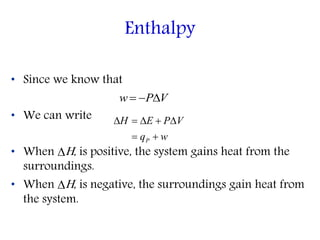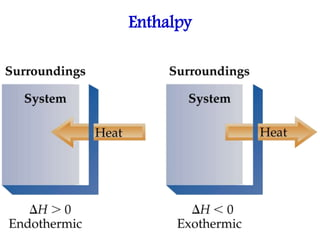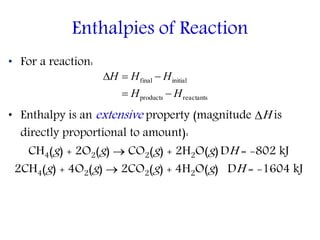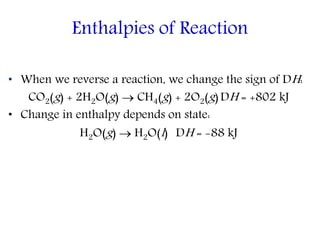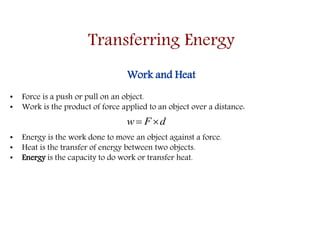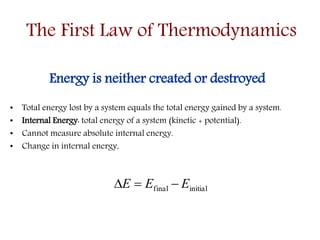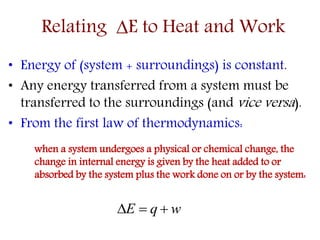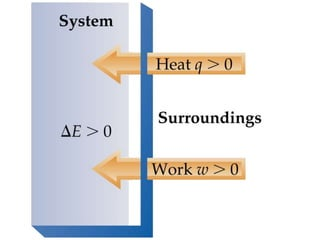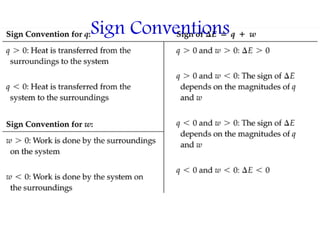1 von 28
Anzeige

### Chapter5

1. Chapter 5 Thermochemistry
2. Kinetic Energy and Potential Energy • Energy is usually defi ned as the capacity to do work • Kinetic energy is the energy of motion: • Potential energy is the energy an object possesses by virtue of its position. • Potential energy can be converted into kinetic energy. Example: a bicyclist at the top of a hill. • Electrostatic potential energy, Ed, is the attraction between two oppositely charged particles, Q1 and Q2, a distance d apart: • The constant  = 8.99  109 J-m/C2. • If the two particles are of opposite charge, then Ed is the electrostatic attraction between them. 2 2 1 mvEk  The Nature of Energy d QQ Ed 21 
3. Units of Energy • SI Unit for energy is the joule, J: • We sometimes use the calorie instead of the joule: 1 cal = 4.184 J (exactly) • A nutritional Calorie: 1 Cal = 1000 cal = 1 kcal    J1m/s-kg1 m/s1kg2 2 1 2 1 2 22   mvEk
4. Heat Capacity and Specific Heat • Calorimetry = measurement of heat flow. • Calorimeter = apparatus that measures heat flow. • Heat capacity = the amount of energy required to raise the temperature of an object (by one degree). • Molar heat capacity = heat capacity of 1 mol of a substance. • Specific heat = specific heat capacity = heat capacity of 1 g of a substance.     if TTT Tq   substanceofgramsheatspecific Calorimetry
5. • Atmospheric pressure is constant! Constant Pressure Calorimetry     T qq   solutionofgrams solutionofheatspecificsolnrxn
6. Constant Pressure Calorimetry
7. • Reaction carried out under constant volume. • Use a bomb calorimeter. • Usually study combustion. Bomb Calorimetry (Constant Volume Calorimetry) TCq  calrxn Example 5.1
8. Systems and Surroundings • Analyzing Energy Changes System: part of the universe we are interested in. Surroundings: the rest of the universe.
9. Types of systems A. open: exchange of matter and energy - example: living systems E0, m0 B. closed: exchange of energy, no exchange of matter – Example: photochemical batch reactor. E0, m=0 C. isolated: no interaction at all with surroundings- - Example: Dewar flask with absolutely rigid walls in a Faraday cage on a space station idealization, but useful E=0, m=0
10. Thermochemical Equations • Enthalpy, H: Heat transferred between the system and surroundings carried out under constant pressure. • Enthalpies of reactions • H = Hproducts - Hreactants
11. Exothermic and Endothermic Processes a. Endothermic: absorbs heat from the surroundings. • An endothermic reaction feels cold.
12. Thermochemical Equations b. Exothermic: transfers heat to the surroundings. • An exothermic reaction feels hot.
13. State Functions • State function: depends only on the initial and final states of system, not on how the internal energy is used.
14. The Law of Hess • When reactants are converted to products, the change in enthalpy is the same whether the reaction takes place in one step or in a series of steps. • Hess’s law is based on the fact that because H is a state function, H depends only on the initial and final state (that is, only on the nature of reactants and products). • The enthalpy change would be the same whether the overall reaction takes place in one step or many steps. • Example 5.3, page 98
15. Enthalpies of formation
16. • Chemical reactions can absorb or release heat. • However, they also have the ability to do work. • For example, when a gas is produced, then the gas produced can be used to push a piston, thus doing work. Zn(s) + 2H+(aq)  Zn2+(aq) + H2(g) • The work performed by the above reaction is called pressure-volume work. • When the pressure is constant, Work VPw 
17. • Enthalpy, H: Heat transferred between the system and surroundings carried out under constant pressure. • Enthalpy is a state function. • If the process occurs at constant pressure, Enthalpy PVEH    VPE PVEH  
18. • Since we know that • We can write • When H, is positive, the system gains heat from the surroundings. • When H, is negative, the surroundings gain heat from the system. Enthalpy VPw  wq VPEH P  
19. Enthalpy
20. • For a reaction: • Enthalpy is an extensive property (magnitude H is directly proportional to amount): CH4(g) + 2O2(g)  CO2(g) + 2H2O(g)DH = -802 kJ 2CH4(g) + 4O2(g)  2CO2(g) + 4H2O(g) DH = -1604 kJ Enthalpies of Reaction reactantsproducts initialfinal HH HHH  
21. • When we reverse a reaction, we change the sign of DH: CO2(g) + 2H2O(g)  CH4(g) + 2O2(g)DH = +802 kJ • Change in enthalpy depends on state: H2O(g)  H2O(l) DH = -88 kJ Enthalpies of Reaction
22. Transferring Energy Work and Heat • Force is a push or pull on an object. • Work is the product of force applied to an object over a distance: • Energy is the work done to move an object against a force. • Heat is the transfer of energy between two objects. • Energy is the capacity to do work or transfer heat. dFw 
23. The First Law of Thermodynamics Energy is neither created or destroyed • Total energy lost by a system equals the total energy gained by a system. • Internal Energy: total energy of a system (kinetic + potential). • Cannot measure absolute internal energy. • Change in internal energy, initialfinal EEE 
24. • Energy of (system + surroundings) is constant. • Any energy transferred from a system must be transferred to the surroundings (and vice versa). • From the first law of thermodynamics: when a system undergoes a physical or chemical change, the change in internal energy is given by the heat added to or absorbed by the system plus the work done on or by the system: Relating E to Heat and Work wqE 
25. Sign Conventions
Anzeige